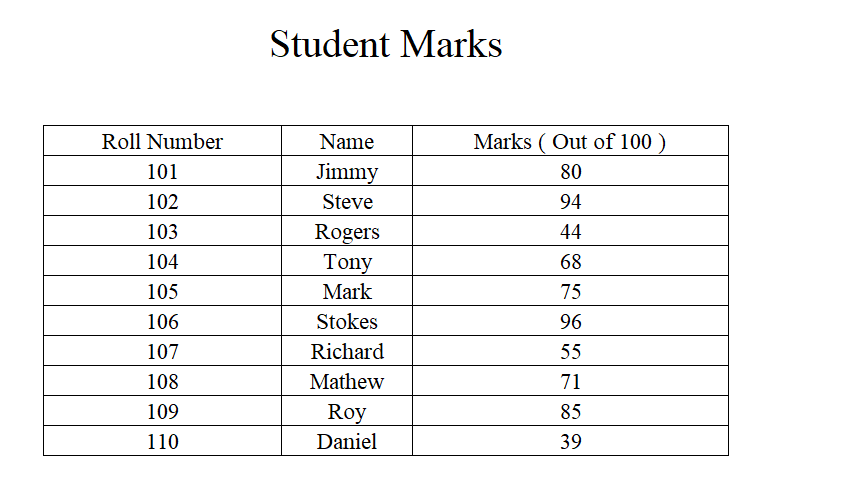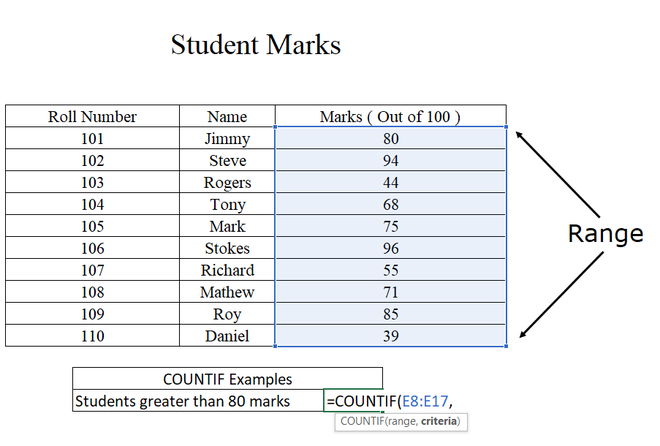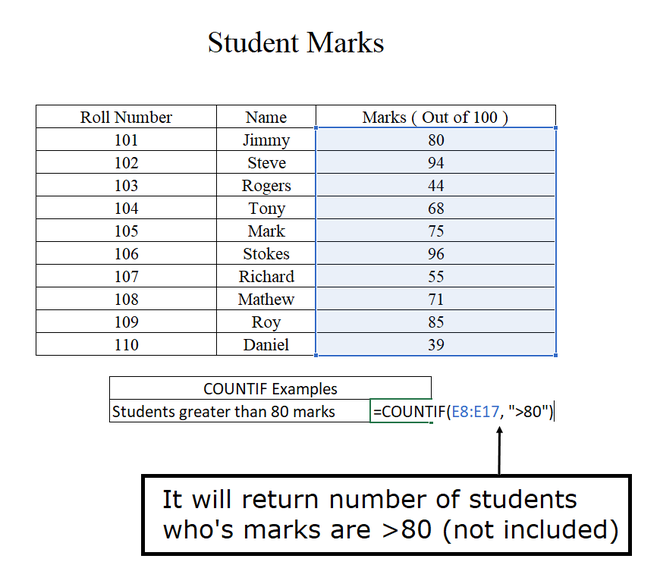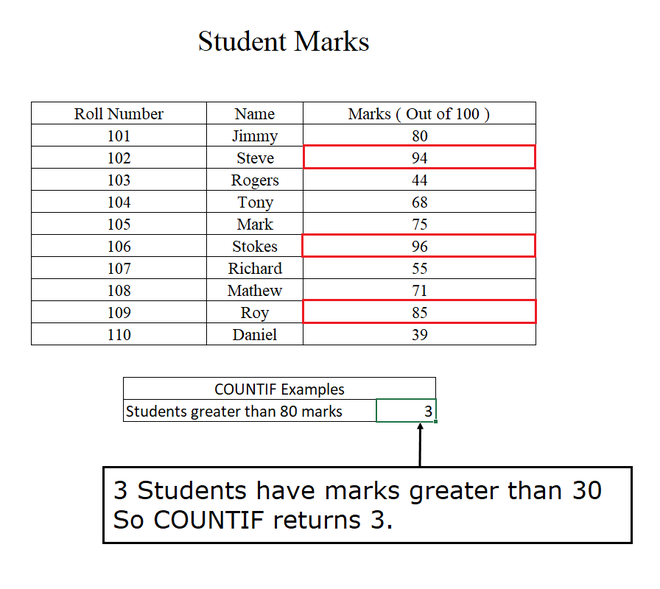# COUNTIF Function in Excel with Examples

• Last Updated : 09 May, 2021

COUNTIF function in excel is used to count the number of cells that match a single condition applied. It can include Dates, Numbers, and Texts. It uses various logical operators like <(Less Than), >(Greater Than), >=(Greater Than or Equal to), <=(Less Than or Equal to), =(Equals to), and <>(NOT) for matching the condition. It also uses some wildcard like * and ? for partial matching.

COUNTIF function in excel is used to count the number of cells.

```Syntax: COUNTIF ( range, criteria)

Arguments:
1. range:- Here ranges refers to the range of cells for which you want the cell count for
a specific condition.
2. criteria:- Here criteria refers to the condition for which you want the cell count.

Return Value: COUNTIF function in excel returns an integer value of the number of cells
which satisfy the given condition.```

Example 1:

Consider the student’s marks which are given below for and XYZ examination.Understanding the arguments of the COUNTIF function in ExcelUnderstanding the argument of the COUNTIF function in Excel

Output:

Note: RED color cells are used for the reference that which cells would satisfy the given criteria. RED color doesn’t come in the output of COUNTIF.The output of the marks of the students having greater than 80

Example 2:

Examples 3:

Some of the other examples are as follows for the COUNTIF function in excel.

We can find out that how many blank cells are there in the given range and also we can find out which are not blank cells in the given range.

• For finding number of Blank cells:   = COUNTIF ( D1:D10, “” )
• For finding a number of not Blank cells: = COUNTIF ( D1:D10, “<>”)

We can use the COUNTIF criteria using the value from another cell using concatenation. In the below example it COUNTIF function will return the value which is equal to the cell E5. Here & is used for the concatenation.

`= COUNTIF ( D1:D10, "=" & E5)`

We can use the COUNTIF function with the DATE function also for finding the dates less than or more than the given DATE. The below example shows how to use the COUNTIF function with the DATE function. It will return some dates that are greater than 1-Jan-2021.

`= COUNTIF ( D1:D10, ">" & DATE( 2021, 1, 1) )`

### Some Points to be remembered:

• COUNTIF is not case sensitive.
• COUNTIF will only check one condition at a time.
• COUNTIF needs a range for the evaluation.
• In COUNTIF the criteria must be enclosed in the double-quotes.
• If the criteria are evaluated from a different cell then that cell must not be enclosed in the double-quotes. E.g. “=” & E5
• It gives an error if the matching string exceeds 255 characters.

My Personal Notes arrow_drop_up